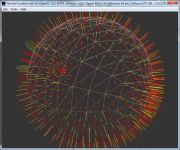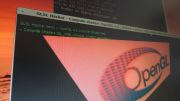Home Utilities Tutorials 3D Demos Graphics Cards Forums About

 GeeXLab
Current version: 0.29.17
>GeeXLab homepage

 FurMark
Current version: 1.21.1
>FurMark homepage

 GPU Caps Viewer
Current version: 1.44.2.1
>GPU Caps Viewer homepage

 GPU Shark
Current version: 0.16.1.0
>GPU Shark homepage

 Blogs
>JeGX's HackLab

 Geeks3D's Articles
>GPU Memory Speed Demystified

>How to Get your Multi-core CPU Busy at 100%

>How To Make a VGA Dummy Plug

>Night Vision Post Processing Filter

 PhysX FluidMark
Current version: 1.5.4
>FluidMark homepage

 TessMark
Current version: 0.3.0
>TessMark homepage

Current version: 0.3.0

 Demoniak3D
Current Version: 1.23.0
>Demoniak3D
>Libraries and Plugins
>Demos
>Codes Samples

3D Graphics Search Engine:The Mandelbrot Set: Colors of Infinity

By Nathan Reed - www.reedbeta.com
and
Jérôme Guinot - www.oZone3D.Net

Initial draft: January 25, 2006

[ Index ]

»Next Page

4 - Mandelbrot on the GPUThe classical algorithm for a Mandelbrot renderer has been known for decades, and most graphics enthusiasts have at one time or another written one.  Today, however, is the era of the Graphics Processing Unit.  Instead of rendering fractals on the CPU, we can exploit the greater power and parallelism of a GPU to make fractal rendering much faster.  Let's see how we can adapt the above algorithm to the GPU.

## 4.1 - A First Attempt

Fortunately for us, the algorithm for rendering the Mandelbrot set is on a per-pixel basis.  Each pixel is processed the same way and independently of all the others.  This means we can simply render a quad that covers the whole screen, and write a fragment shader to perform the iteration process:

```uniform vec4 insideColor;
uniform sampler1D outsideColorTable;
uniform float maxIterations;
void main ()
{
vec2 c = gl_TexCoord.xy;
vec2 z = c;
gl_FragColor = insideColor;
for (float i = 0; i < maxIterations; i += 1.0)
{
z = vec2(z.x*z.x - z.y*z.y, 2.0*z.x*z.y) + c;
if (dot(z, z) > 4.0)
{
gl_FragColor = texture1D(outsideColorTable,
i / maxIterations);
break;
}
}
}```

This is precisely the same algorithm as was presented above; it has been rewritten in GLSL (OpenGL Shading Language).It assumes that you have set up the color table as a 1D texture map in outsideColorTable and that the texture coordinates passed to the shader correspond to complex numbers.  Then the shader calculates the sequence using complex multiplication and checks each value to see if it escapes from the radius-2 circle.

Unfortunately, this shader will be very slow, and you will not be able to render the fractal in real-time, unless maxIterations is set very low.  Moreover, the looping and branching inside the shader means that it will not even run on graphics hardware that does not support SM 3.0.  At the time of this writing, you need a GeForce 6600 or better GPU to even execute the above shader, and it would still be very slow even on a GeForce 7800, currently the most powerful card in the consumer market.

## 4.2 - Stream Processing

Instead of performing the Mandelbrot iteration in a single complicated fragment shader, a better approach is to use a multipass algorithm.  To implement this, we can use the stream processing model of general purpose GPU computation.  One shader generates a set of data, which is then used as input to another shader; the output of the second shader is the input to a third, and so forth.  Since shaders cannot operate "in place," i.e. they cannot write to the same framebuffer from which they are reading, we must use a minimum of two buffers.  We then ping-pong between these buffers, first using one as the input and the other as the output, then switching their roles for the next shader pass.

To render the Mandelbrot set in a multipass algorithm, we will use floating-point framebuffers to store the value of zn at each pixel.  Three shaders in total are used; the first sets up the initial values, by simply storing the texture coordinates (which represent the values of c, which equals z1) to the red and green components of each pixel:

```void main ()
{
vec2 c = gl_TexCoord.xy;
gl_FragColor = vec4(c, 0, 0);
}```

The second shader will be executed over and over again.  This shader actually performs the iteration, calculating the next value of zn at each pixel.  In this shader, we get the previous value zn-1 by sampling a texture, which is the output of the previous pass; the value of c is provided by texture coordinates as before.

```uniform sampler2D input;
uniform float curIteration;
void main ()
{
// Lookup value from last iteration
vec4 inputValue = texture2D(input, gl_TexCoord.xy);
vec2 z = inputValue.xy;
vec2 c = gl_TexCoord.xy;

// Only process if still within radius-2 boundary
if (dot(z, z) > 4.0)
// Leave pixel unchanged (but copy
//through to destination buffer)
gl_FragColor = inputValue;
else
{
gl_FragColor.xy = vec2(z.x*z.x - z.y*z.y, 2.0*z.x*z.y) + c;
gl_FragColor.z = curIteration;
gl_FragColor.w = 0.0;
}
}```

As you can see, we are also storing n (the number of the current iteration, passed in as a uniform variable), in the blue component of the pixel.  This is of use in the third shader, which is used for finally displaying the fractal.  Its input is the floating-point buffer containing the final zn values, but its output is an ordinary color buffer.

```uniform sampler2D input;
uniform vec4 insideColor;
uniform sampler1D outsideColorTable;
uniform float maxIterations;
void main ()
{
// Lookup value from last iteration
vec4 inputValue = texture2D(input, gl_TexCoord.xy);
vec2 z = inputValue.xy;

if (dot(z, z) > 4.0)
gl_FragColor = texture1D(outsideColorTable,
inputValue.z / maxIterations);
else
gl_FragColor = insideColor;
}```

This three-shader multipass algorithm can render the same image as our original shader, but will run on any hardware that supports floating-point buffers.  In the ATI world, this includes the Radeon 9500 and up, and in nVIDIA hardware the GeForce 5200 and up—much more reasonable expectations.  (Note that although two of the shaders use if statements, on pre-SM 3.0 cards this will produce a CMP instruction rather than an actual branch.)

The shaders presented above can be made to work together in a variety of different ways.  The most obvious is to use the iterating shader a few times on off-screen surfaces and then display the result.  However, a cool effect can be created by running the viewing shader after each iteration.  The fractal animates, appearing to grow inward as more and more detail is revealed.  Another possibility is to map the fractal onto a 3D surface, which can be done as easily as ordinary texture mapping, simply using the viewing shader to draw the triangles of the surface.

Two problems still remain unsolved in our improved GPU Mandelbrot renderer, though.  First, there is no antialiasing on the fractal, since it is essentially point-sampled.  This makes the generated images look rather ugly, unless they are rendered at a high resolution and then downsampled.  The second problem is precision.  Currently, ATI cards use a 24-bit floating-point format and nVIDIA cards a 32-bit format for the registers in the shading units, and the floating-point buffers also store only 32-bit floats.  This means that one cannot zoom very far into the fractal before precision breaks down; one can get to about 10-7 with 32-bit floats and only 10-5 with 24-bit floats.  This is currently an unavoidable limitation of GPU hardware; CPU-based Mandelbrot renderers use 64-bit floats or even provide their own implementations of arbitrary-precision floating-point arithmetic.

[ Index ]

»Next Page

 GeeXLab demosGLSL - Mesh exploderPhysX 3 cloth demoNormal visualizer with GS